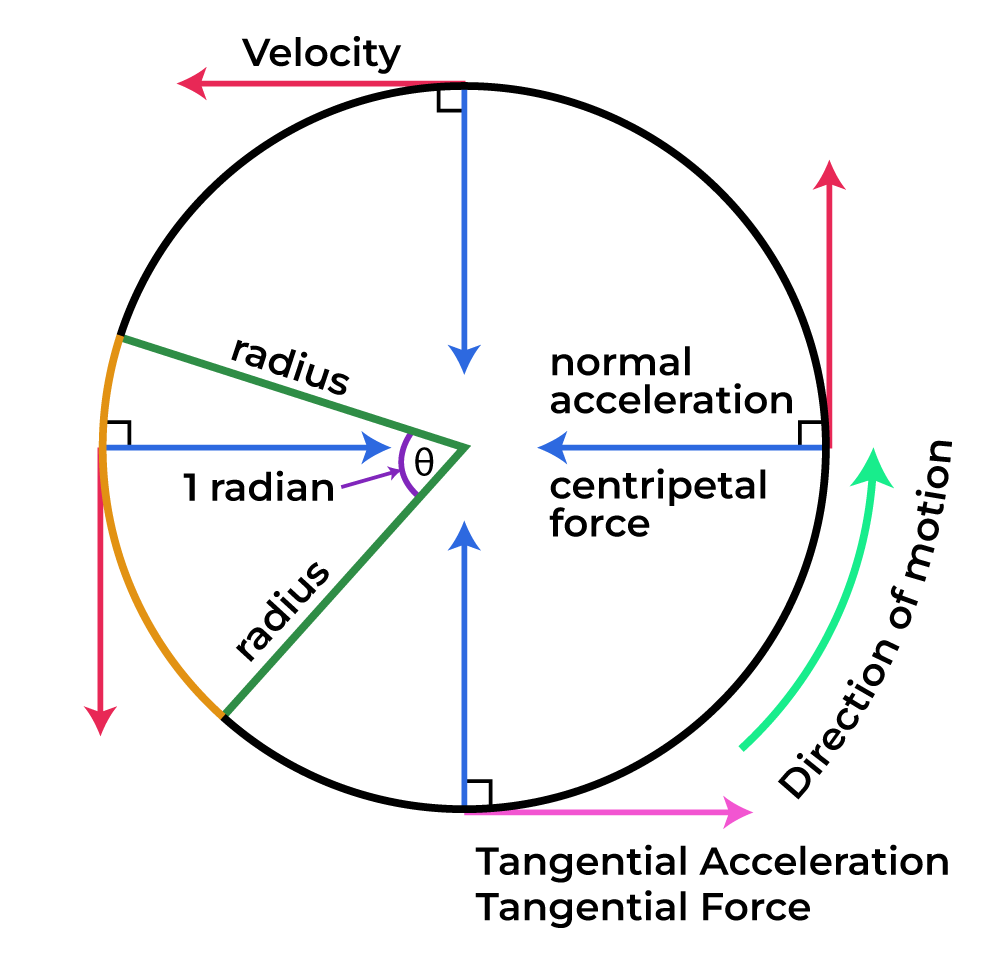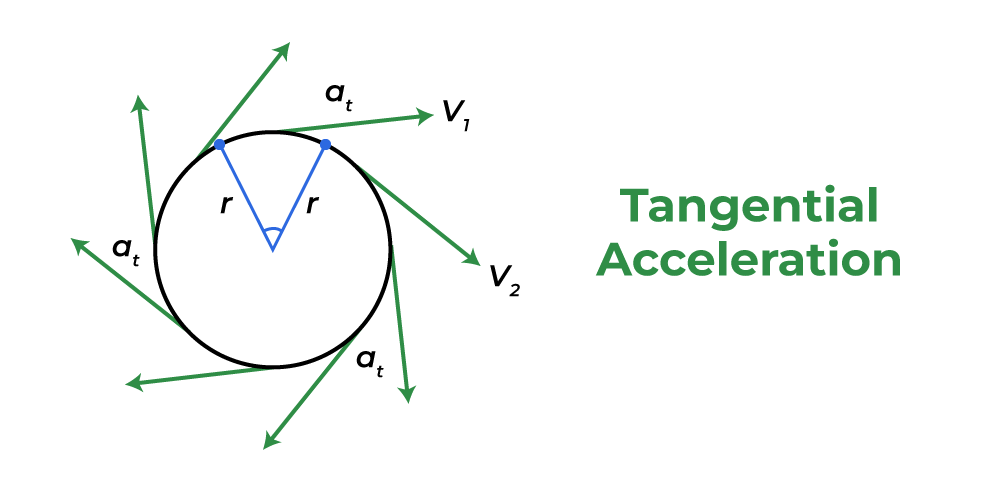Open in App
Not now

# Tangential Acceleration Formula

• Last Updated : 19 Dec, 2022

Tangential acceleration is the rate at which a tangential velocity varies in the rotational motion of any object. It acts in the direction of a tangent at the point of motion for an object. The tangential velocity also acts in the same direction for an object undergoing circular motion. Tangential acceleration only exists when an object travels in a circular path. It is positive if the body is rotating at a faster velocity, negative when the body is decelerating, and zero when the body is moving uniformly in the orbit.## Tangential Acceleration

Tangential acceleration is similar to linear acceleration, however, it is only in one direction. This has something to do with circular motion. Tangential acceleration is therefore the rate of change of a particle’s tangential velocity in a circular orbit. It always points to the tangent of the body’s route.

Tangential acceleration works when an object moves in a circular path. Tangential acceleration is similar to linear acceleration, but it is not the same as straight-line linear acceleration. If an item moves in a straight line, it is linearly accelerating.A car, for example, speeding around a bend in the road. The automobile is accelerating tangentially to the path’s bend.

## Tangential Acceleration Formula

The tangential acceleration is denoted by the symbol at. Its unit of measurement is the same as linear acceleration, that is, meters per square second (m/s2). Its dimensional formula is given by [M0L1T-2]. Its formula is given by the product of the radius of a circular path and the angular acceleration of the rotating object.

at = r α

where,

• at is the tangential acceleration,
• r is the radius of circular path,
• α is the angular acceleration.

The above expression gives the relation between tangential acceleration and angular acceleration.

Now, in terms of angular velocity and time, the formula is given by,

at = r (ω/t)

where,

• at is the tangential acceleration,
• ω is the angular velocity,
• t is the time taken.

In terms of angular displacement and time, the formula is given by,

at = r (θ/t2)

where,

• at is the tangential acceleration,
• θ is the angular displacement or angle of rotation,
• t is the time taken.

Following are the various cases possible for different values of Tangential Acceleration:

1. When at is greater than Zero: The object has an accelerated motion, and the magnitude of velocity will increase with time.
2. When at is Less than Zero: The object has a deaccelerated or slow motion, and the magnitude of velocity will decrease with time.
3. When at is Equal to Zero: The object has a uniform motion, and the magnitude of velocity will remain constant.

## Solved Examples on Tangential Acceleration

Example 1: Calculate the tangential acceleration if an object is undergoing circular motion for radius 5 m and angular acceleration 2 rad/s2

Solution:

We have,

r = 5

α = 2

Using the formula we get,

at = r α

= 5 (2)

= 10 m/s2

Example 2: Calculate the tangential acceleration if an object is undergoing circular motion for a radius of 12 m and angular acceleration of 0.5 rad/s2.

Solution:

We have,

r = 12

α = 0.5

Using the formula we get,

at = r α

= 12 (0.5)

= 6 m/s2

Example 3: Calculate the angular acceleration if an object is undergoing circular motion for radius 20 m and tangential acceleration 40 m/s2.

Solution:

We have,

r = 20

at = 40

Using the formula we get,

at = r α

α = at/r

= 40/20

Example 4: Calculate the angular acceleration if an object is undergoing circular motion for radius 2 m and tangential acceleration 20 m/s2.

Solution:

We have,

r = 2

at = 20

Using the formula we get,

at = r α

α = at/r

= 20/2

Example 5: Calculate the radius if an object is undergoing circular motion for an angular acceleration of 4 rad/s2 and tangential acceleration of 20 m/s2.

Solution:

We have,

α = 4

at = 20

Using the formula we get,

at = r α

r = at

= 20/4

= 5 m

## FAQs on Tangential Acceleration

Question 1: What are the values of radial and tangential acceleration when the motion of a particle is uniformly accelerated?

Even though there is no tangential acceleration, the centripetal acceleration must be present to alter the direction of velocity at all times, and the centripetal acceleration is the net acceleration in this instance. This is an example of uniform circular motion.

Thus, If ar and at represent radial and tangential acceleration then, ar ≠ 0 and at = 0.

Question 2: What is Tangential Acceleration?

Tangential acceleration is the rate at which a tangential velocity varies in the rotational motion of any object. It acts in the direction of a tangent at the point of motion for an object.

Question 3: What is the value of Tangential Acceleration in Uniform Circular Motion?

The tangential acceleration is zero for uniform circular motion. In a uniform circular motion, angular velocity remains constant thus tangential acceleration = 0.

Question 4: What is the SI Unit of Tangential Acceleration?

The SI Unit of Tangential Acceleration is m/s2.

Question 5: What is the relation between Tangential Acceleration and Angular Acceleration?

The Tangential Acceleration formula is given by the product of the radius of a circular path and the angular acceleration of the rotating object.

at = r α

where,

• at is the tangential acceleration,
• r is the radius of circular path,
• α is the angular acceleration.

My Personal Notes arrow_drop_up